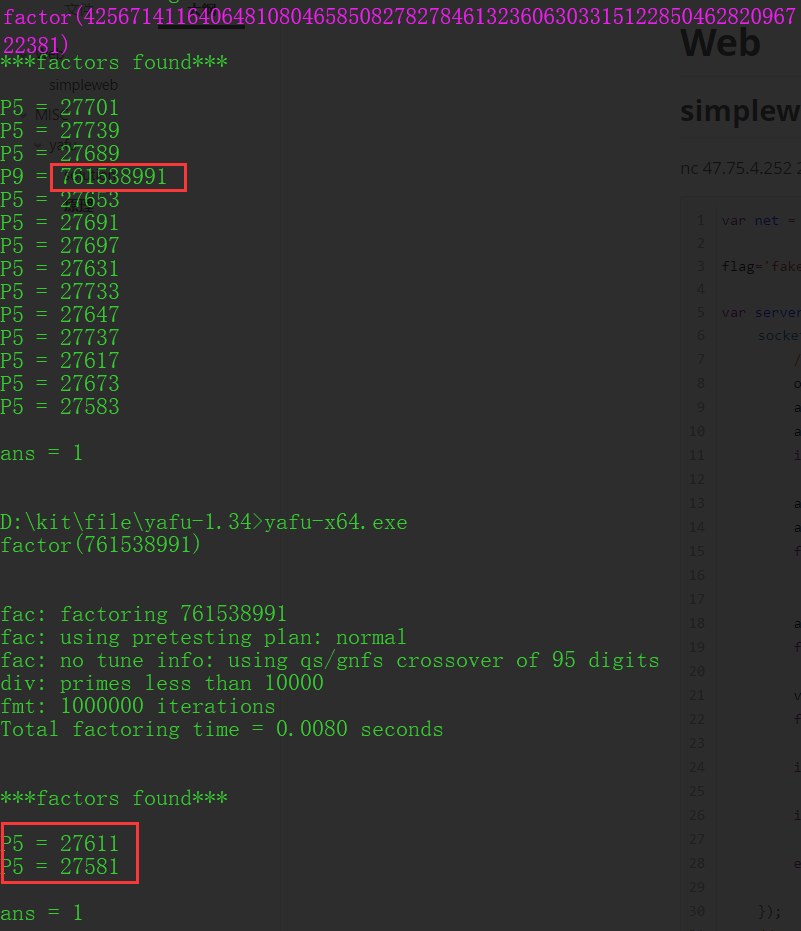# Web#

## simpleweb#

nc 47.75.4.252 23333

 `````` 1 2 3 4 5 6 7 8 9 10 11 12 13 14 15 16 17 18 19 20 21 22 23 24 25 26 27 28 29 30 31 32 33 34 35 36 37 38 `````` ``````var net = require('net'); flag='fake_flag'; var server = net.createServer(function(socket) { socket.on('data', (data) => { //m = data.toString().replace(/[\n\r]*\$/, ''); ok = true; arr = data.toString().split(' '); arr = arr.map(Number); if (arr.length != 5) ok = false; arr1 = arr.slice(0); arr1.sort(); for (var i=0; i<4; i++) if (arr1[i+1] == arr1[i] || arr[i] < 0 || arr1[i+1] > 127) ok = false; arr2 = [] for (var i=0; i<4; i++) arr2.push(arr1[i] + arr1[i+1]); val = 0; for (var i=0; i<4; i++) val = val * 0x100 + arr2[i]; if (val != 0x23332333) ok = false; if (ok) socket.write(flag+'\n'); else socket.write('nope\n'); }); //socket.write('Echo server\r\n'); //socket.pipe(socket); }); HOST = '0.0.0.0' PORT = 23333 server.listen(PORT, HOST); ``````

 `````` 1 2 3 4 5 6 7 8 9 10 11 12 13 14 15 16 17 18 19 20 21 22 23 24 25 26 27 28 29 30 31 32 33 34 35 36 37 38 39 40 `````` ``````# understand JavaScript Code # var arr = [22,12,3,43,56,47,4]; # arr.sort(); # console.log(arr);//[12, 22, 3, 4, 43, 47, 56] # console.log(arr.slice(0));//[12, 22, 3, 4, 43, 47, 56] u=128 def aLSSb(a,b): return str(a)

# MISC#

## yafu#

nc 47.75.4.252 10004

 `````` 1 2 3 4 5 6 7 8 9 10 11 12 13 14 15 16 17 18 19 20 21 22 23 24 25 26 27 28 29 30 31 32 33 34 35 36 37 38 39 40 41 42 43 44 45 46 47 48 49 50 51 52 53 54 55 56 57 58 59 60 61 62 63 64 65 66 67 68 69 70 71 72 73 74 75 76 77 78 79 80 81 82 `````` ``````#!/usr/bin/python3 import random,sys import socketserver import binascii import os import hmac,hashlib from hashlib import sha256 from Crypto.Util.number import isPrime import string from subprocess import run, PIPE, TimeoutExpired from flag import FLAG basedir = '/home/sixstars/*ctf/yafu/yafu-1.34' cmd = basedir+'/yafu' timelimit = 2 def do_init(): url = 'https://sourceforge.net/projects/yafu/files/1.34/yafu-1.34.zip/download' if os.path.isfile(cmd): return os.system("wget %s -O %s/yafu-1.34.zip" % (url, basedir)) os.system("unzip %s/yafu-1.34.zip -d %s/" % (basedir, basedir)) os.system("chmod +x %s" % (cmd)) def do_factor(num): try: res = run(cmd, stdout=PIPE, input=('factor(%d)'%num).encode(), timeout=timelimit) except TimeoutExpired: return False tmp = res.stdout.decode() tmp = tmp[tmp.find('***factors found***\n\n')+21:].split('\n') for line in tmp: pos = line.find(' = ') if pos == -1: break factor = int(line[pos+3:]) if line == 'P' and not isPrime(factor): return True return False class Task(socketserver.BaseRequestHandler): def proof_of_work(self): proof = ''.join([random.choice(string.ascii_letters+string.digits) for _ in range(20)]) print(proof) digest = sha256(proof.encode('ascii')).hexdigest() self.request.send(str.encode("sha256(XXXX+%s) == %s\n" % (proof[4:],digest))) self.request.send(str.encode('Give me XXXX:')) x = self.request.recv(10).decode() x = x.strip() wtfpy3 = x+proof[4:] if len(x) != 4 or sha256(wtfpy3.encode('ascii')).hexdigest() != digest: return False return True def handle(self): if not self.proof_of_work(): return do_init() self.request.sendall(str.encode("No bruteforce required. Do not DOS plz >_<\n")) self.request.sendall(str.encode("The number to factorize: ")) x = self.request.recv(1024) try: x = int(x, 16) except: return if do_factor(x): self.request.sendall(str.encode("What happened? Anyway your flag here: %s\n" % FLAG)) else: self.request.sendall(str.encode("Bye\n")) class ThreadedServer(socketserver.ThreadingMixIn, socketserver.TCPServer): pass if __name__ == "__main__": HOST, PORT = '0.0.0.0', 10004 print(HOST) print(PORT) server = ThreadedServer((HOST, PORT), Task) server.allow_reuse_address = True server.serve_forever() ``````

### solution#

 `````` 1 2 3 4 5 6 7 8 9 10 11 12 13 14 15 16 17 18 19 20 21 22 23 24 25 26 27 28 29 30 31 32 33 34 35 36 37 38 `````` ``````import socket import re import string import itertools import hashlib def crack_sha256(data_part,hash): for i in itertools.product(string.ascii_letters+string.digits,repeat=4): if hashlib.sha256(''.join(i)+data_part).hexdigest()==hash: return ''.join(i).strip() def get(s): buffer = [] try: while True: d = s.recv(10) if d: buffer.append(d) except Exception as e: data = ''.join(buffer) return data socket.setdefaulttimeout(2) s = socket.socket(socket.AF_INET, socket.SOCK_STREAM) s.connect(('47.75.4.252', 10004)) # ------------ proof_din=get(s) print proof_din r=re.findall("sha256\(XXXX\+([0-9a-zA-Z]{16})\) == ([0-9a-z]{64})",proof_din) # print r proof_part,sha_value=r,r # print proof_part,sha_value proof_part_0=crack_sha256(proof_part,sha_value) s.send(proof_part_0) print "XXXX is %s"%proof_part_0 # ------------ print get(s) s.send('286b7fb2af5f5d27d216771c90ac6f43a9892a690c48e4b06bcbc1cd') print get(s) ``````

### 原理#

When yafu delete factors, it fails to update the factor type correctly. So it’s possible to fool yafu if you use a smooth number which consists of many small primes. Follow the link in `solve.py` for more details.